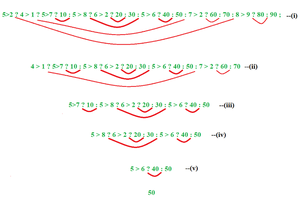Open In App

# C++ | Nested Ternary Operator

Ternary operator also known as conditional operator uses three operands to perform operation.
Syntax :

`op1 ? op2 : op3;`

Nested Ternary operator: Ternary operator can be nested. A nested ternary operator can have many forms like :

• a ? b : c
• a ? b: c ? d : e ? f : g ? h : i
• a ? b ? c : d : e

Let us understand the syntaxes one by one :

1. a ? b : c => This ternary operator is similar to if-else statement. So it can be expressed in form of if-else statement.
Expression using Ternary operator:

`a ? b : c`

Expression using if else statement:

```if ( a )
then b execute
else
c execute```

2.Example:

## C++

 `// C++ program to illustrate``// nested ternary operators``#include ``using` `namespace` `std;``   ` `int` `main()``{``    ``cout << ``"Execute expression using"``    ``<< ``" ternary operator: "``;``    ``// Execute expression using``    ``// ternary operator``    ``int` `a = 2 > 5 ? 2 : 5;``    ``cout << a << endl;``       ` `    ``cout << ``"Execute expression using "``    ``<< ``"if else statement: "``;``       ` `    ``// Execute expression using if else``    ``if` `( 2 > 5)``        ``cout << ``"2"``;``    ``else``        ``cout << ``"5"``;``    ``return` `0;``}`

Output:

```Execute expression using ternary operator: 5
Execute expression using if else statement: 5```

2. a ? b: c ? d : e ? f : g ? h : i =>This Nested ternary operator can be broken into if, else and else-if statement. The expression can break into smaller piece in ternary operator and if else statement which are given below:
Expression using ternary operator:

```a ? b
: c ? d
: e ? f
: g ? h
: i```

Expression using if else statement:

``` if a then b
else if c then d
else if e then f
else if g then h
else i```

## CPP

 `// C++ program to illustrate``// nested ternary operators``#include `` ` `using` `namespace` `std;`` ` `int` `main()``{``    ``cout << ``"Execute expression using "``    ``<< ``"ternary operator: "``;``    ``int` `a = 2 > 3 ? 2 : 3 > 4 ? 3 : 4;``    ``cout << a << endl;``     ` `    ``cout << ``"Execute expression using "``    ``<< ``"if else statement: "``;``    ``if` `( 2 > 3 )``        ``cout << ``"2"``;``    ``else` `if` `( 3 > 4 )``        ``cout << ``"3"``;``    ``else` `        ``cout << ``"4"``;``    ``return` `0;``}`

1.
Output:

```Execute expression using ternary operator: 4
Execute expression using if else statement: 4```

3. a ? b ? c : d : e => Below is the expansion of expression using ternary operator and if else statement.
Expression using ternary operator:

``` a ?
b ? c
: d
: e```

Expression using if else statement:

```if ( a )
if ( b )
c execute
else
d execute
else
e execute```

## CPP

 `// C++ program to illustrate``// nested ternary operators``#include `` ` `using` `namespace` `std;`` ` `int` `main()``{``    ``cout << ``"Execute expression using "``    ``<< ``"ternary operator: "``;``    ``int` `a = 4 > 3 ? 2 > 4 ? 2 : 4 : 3;``    ``cout << a << endl;``     ` `    ``cout << ``"Execute expression using "``    ``<< ``"if else statement: "``;``    ``if` `( 4 > 3 )``        ``if` `( 2 > 4 )``            ``cout << ``"2"``;``        ``else``            ``cout << ``"4"``;``    ``else``        ``cout << ``"3"``;``    ``return` `0;``}`

Output:

```Execute expression using ternary operator: 4
Execute expression using if else statement: 4```

//improved by sathiyamoorthics19

Example 2: Evaluate the following statement.

5 > 2 ? 4 > 1 ? 5>7 ? 10 : 5 > 8 ? 6 > 2 ? 20 : 30 : 5 > 6 ? 40 : 50 : 7 > 2 ? 60 : 70 : 8 > 9 ? 80 : 90 ;

To solve the above problem, Grouping concept must be known.

First, check there is an equal number of colon and question mark.If it is equal, the statement is valid, if not it is an error statement.

1. Come to first colon ( : ) and match the left nearest question mark ( ? )
2. Repeat the process and continue until no colon ( : ) leftKey points :

First question mark ( ? ) to colon ( : )is considered as expression 2 and from that colon (:) to last is considered as expression 3

( i ) 5 > 2 is true, so come to the expression 2 which is question mark ( ? ) to colon ( : )is considered as expression 2 and execute.

( ii ) 4 > 1 is true, so come to the expression 2 and execute.

( iii ) 5 > 7 is false, so come to expression 3 and execute.

( iv ) 5 > 8 is false ,so come to expression 3 and execute.

( v ) 5 > 6 is false again, so come to expression 3 and the answer is 50.

## C

 `#include `` ` `int` `main()``{``    ``int` `result;``    ``result =5>2 ? 4 > 1 ? 5>7 ? 10 : 5 > 8 ? 6 > 2 ? 20 : 30 : 5 > 6 ? 40 : 50 : 7 > 2 ? 60 : 70 : 8 > 9 ? 80 : 90 ; ``    ``printf``(``"Output : %d"``, result);``    ``return` `0;``}`` ` `//improved by sathiyamoorthics19`

Output

`Output : 50`